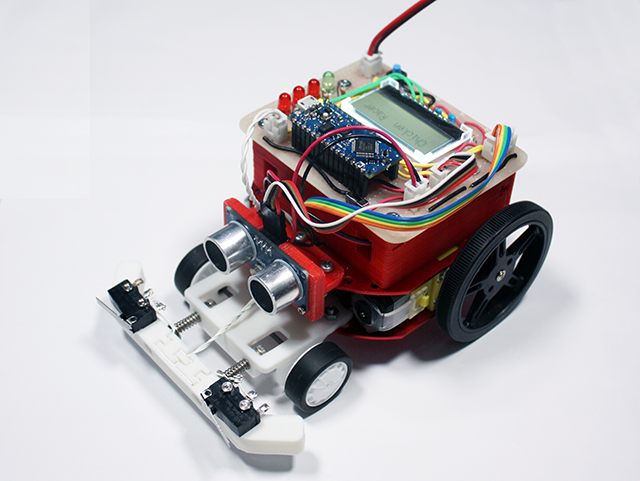## 入门篇：Arduino蓝牙手套第一部分-基础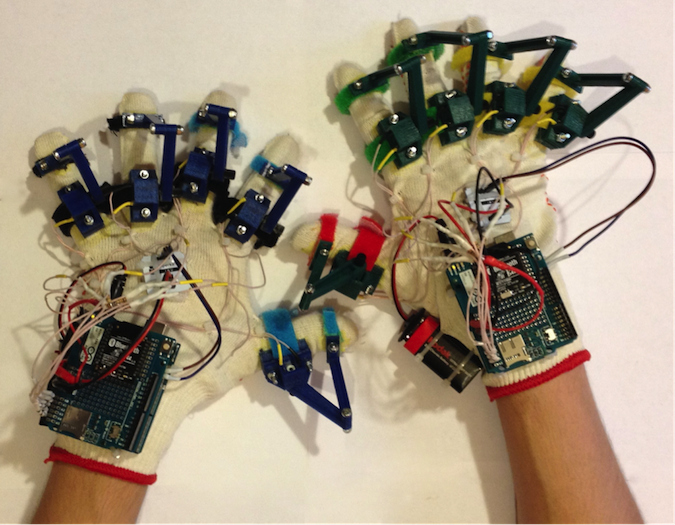## 硬件

• • Arduino UNO
• • 面包板
• • 电位计 0-10kOhm
• • 电阻 10 kOhm
• • IMU 传感器
• • 电线
• • Arduino 无线扩展板
• • Xbee USB 适配器
• • Bluetooth Bee
• • PC 蓝牙适配器
• • USB A-B 数据线
• • 微型 USB 数据线

## 软件

• • Arduino IDE 1.6.7
• • LabVIEW

## 工具

• • 万用表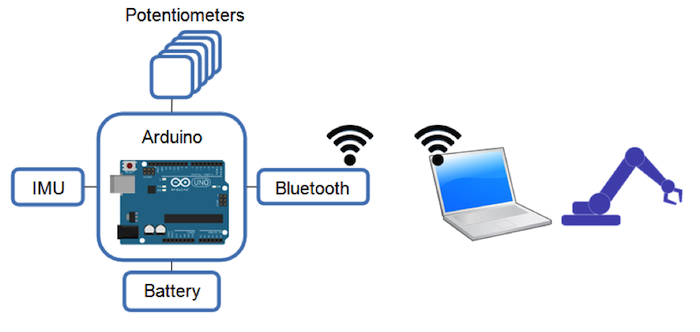## 旋转测量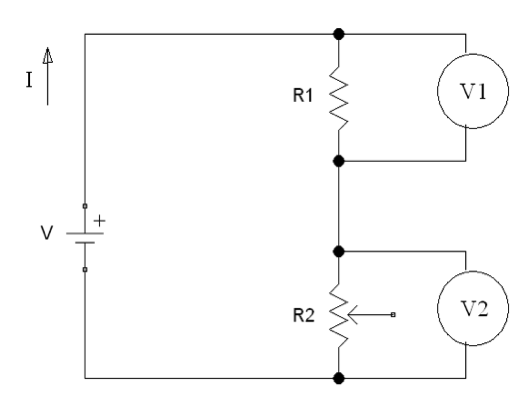V 是来自Arduino 5V电源的电压； I 是 流经电路的电流； R1是具有固定电阻值的电阻； R2 是具有可变电阻的电位计； V1和V2是电压表。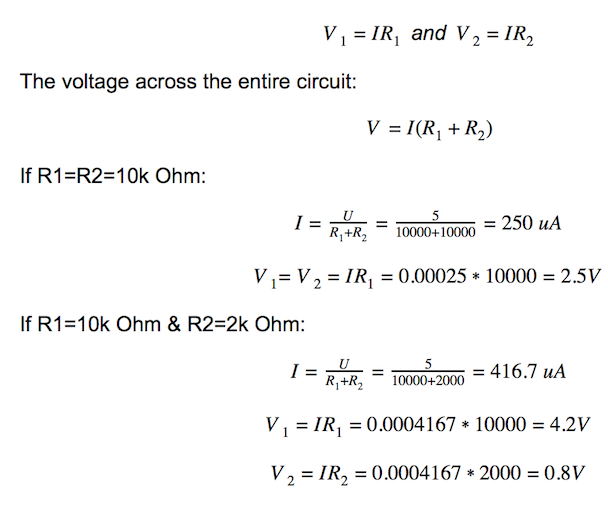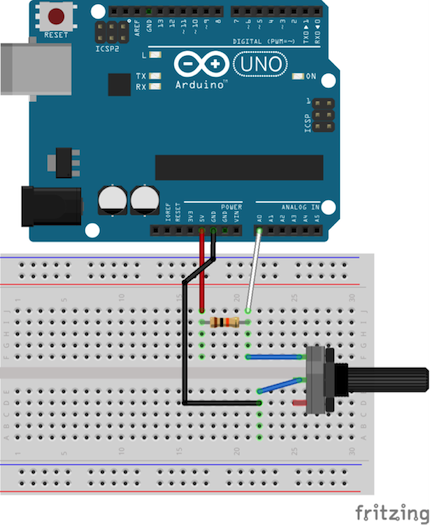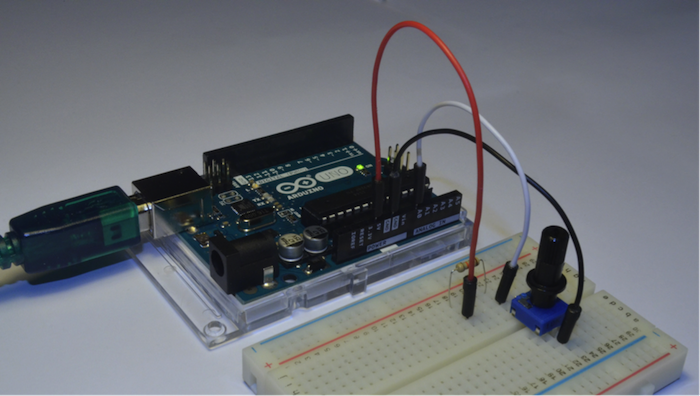``````int sensorValue;
void setup()
{
Serial.begin(9600);// initialize serial communication at 9600 bits per second
}

void loop()
{
Serial.println(sensorValue);// print out the value you read
delay(100);        // delay in between reads for stability
}
``````

## 定向测量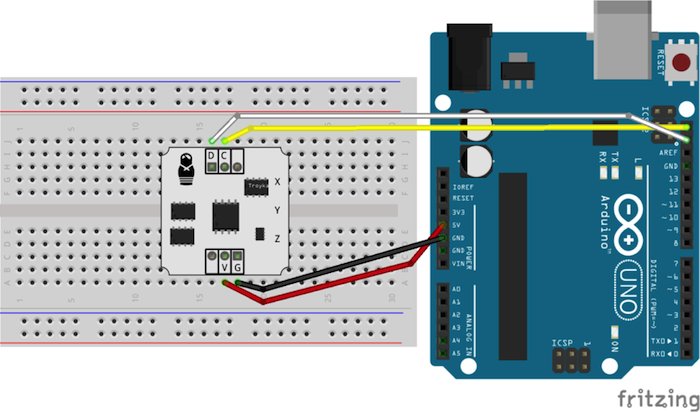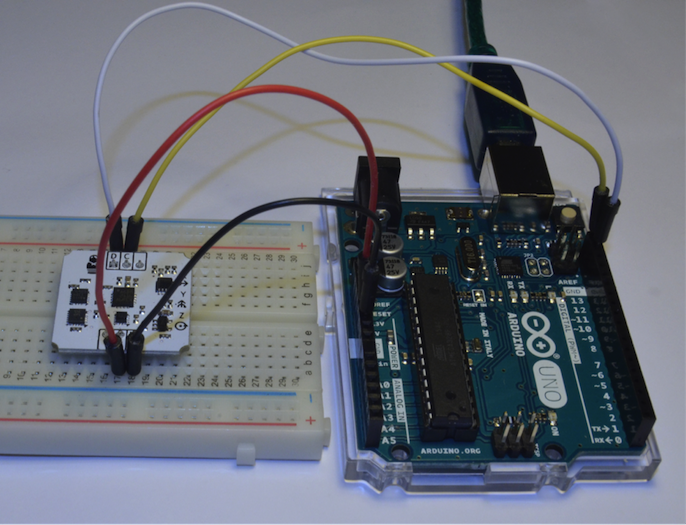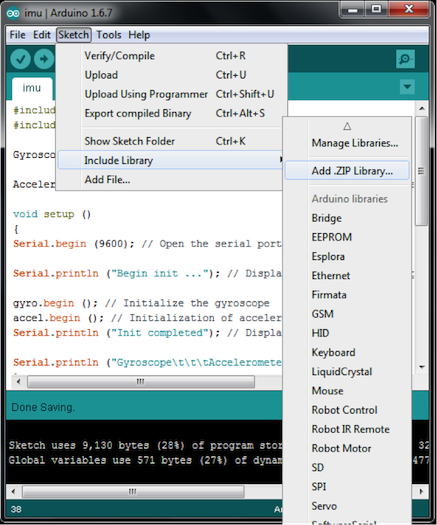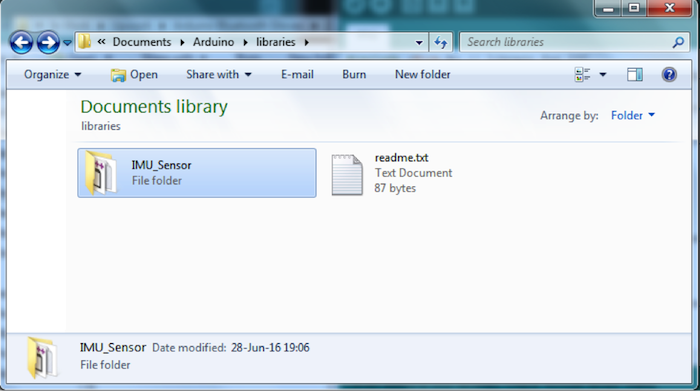``````#include <Wire.h> // Library for I²C
#include <imu.h> // Library for working with IMU modules

Gyroscope gyro; // Create an object to work with Gyroscope

Accelerometer accel; // Create an object to work with Accelerometer

void setup ()
{
Serial.begin (9600); // Open the serial port

Serial.println ("Begin init ..."); // Display a message on the beginning of the initialization

gyro.begin (); // Initialize the gyroscope
accel.begin (); // Initialization of accelerometers
Serial.println ("Init completed"); // Display a message about the successful initialization

Serial.println ("Gyroscope\t\t\tAccelerometer");
}

void loop ()
{
Serial.print (gyro.readX_DegPerSec ()); // Output angular velocity around the axis X
Serial.print ("\t");
Serial.print (gyro.readY_DegPerSec ()); // Output of the angular velocity around the Y axis
Serial.print ("\t");
Serial.print (gyro.readZ_DegPerSec ()); // Output of the angular velocity about the Z axis
Serial.print ("\t\t");

Serial.print (accel.readX_G ()); // Output of the direction and magnitude of acceleration along the X axis
Serial.print ("\t");
Serial.print (accel.readY_G ()); // Output of the direction and magnitude of acceleration along the Y-axis
Serial.print ("\t");
Serial.print (accel.readZ_G ()); // Output of the direction and magnitude of acceleration along the Z axis
Serial.print ("\t\t");

Serial.println ("");
delay (300);
}
``````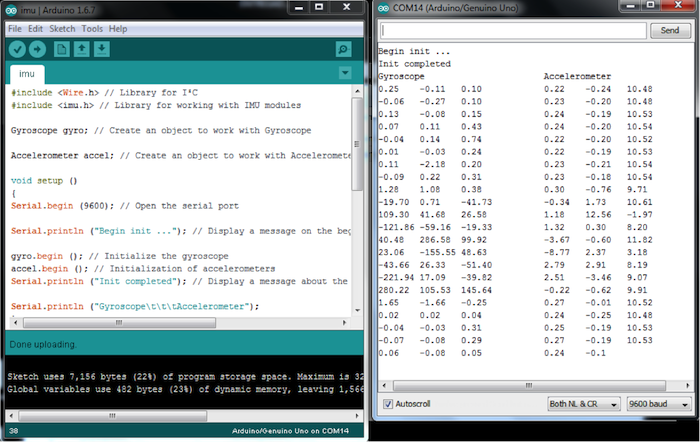## 无线数据传输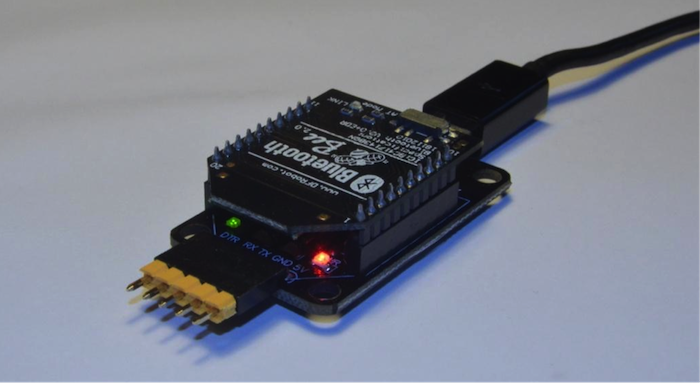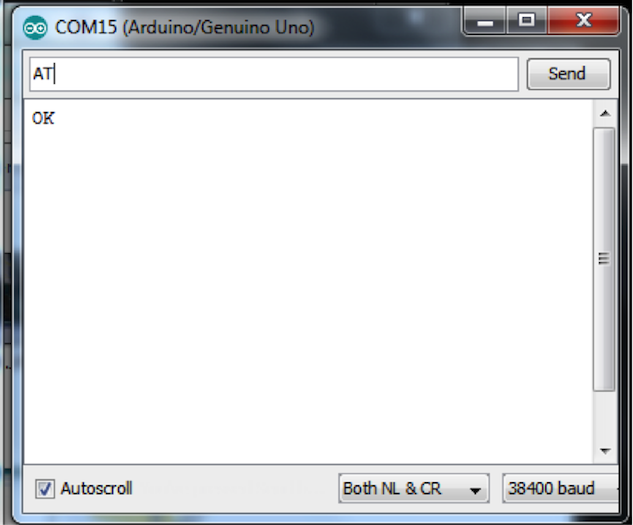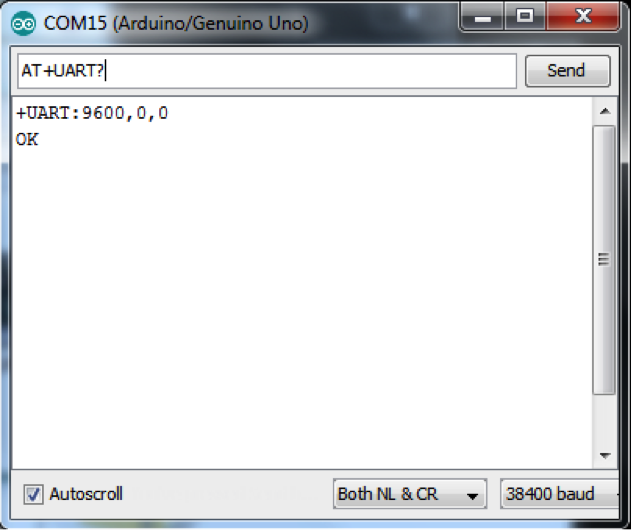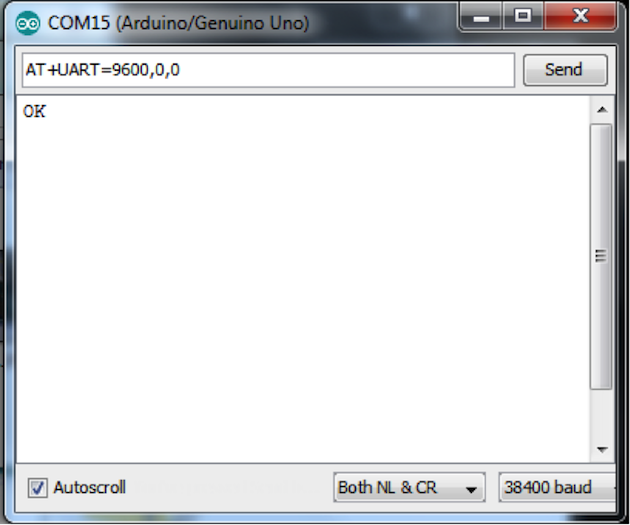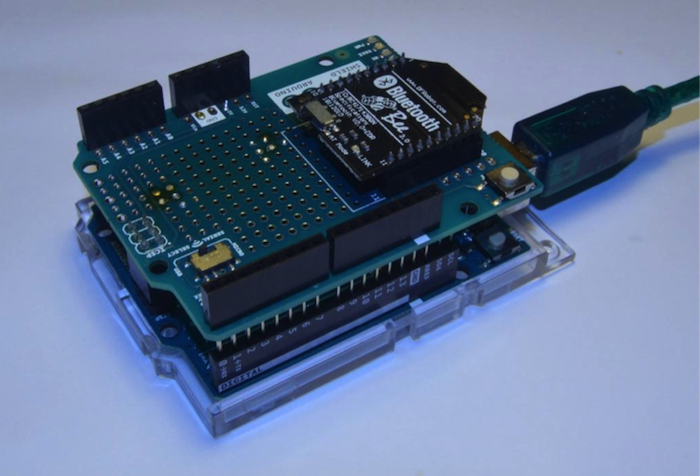``````#define LED 13
int pause = 0;    // variable to store sent value

void setup()
{
pinMode(LED, OUTPUT);
Serial.begin(9600);  // open the serial port
}

void loop()
{
if (Serial.available() > 0) {
Serial.println("Get Message:"+inStr);
pause = inStr.toInt();
}

digitalWrite(LED, HIGH);   // turn the LED on (HIGH is the voltage level)
delay(pause);              // wait for a second
digitalWrite(LED, LOW);    // turn the LED off by making the voltage LOW
delay(pause);              // wait for a second
}
``````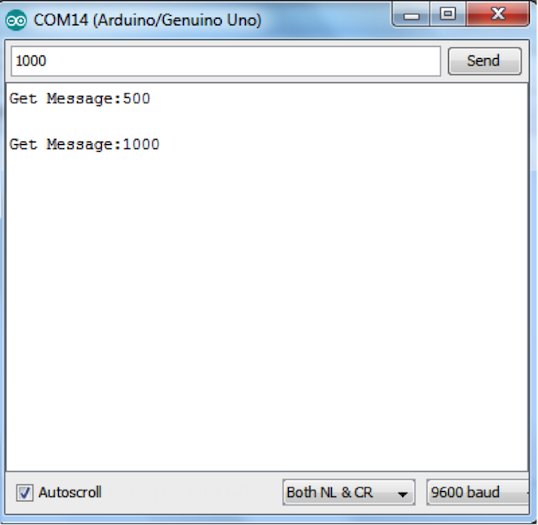### 相关文章

1.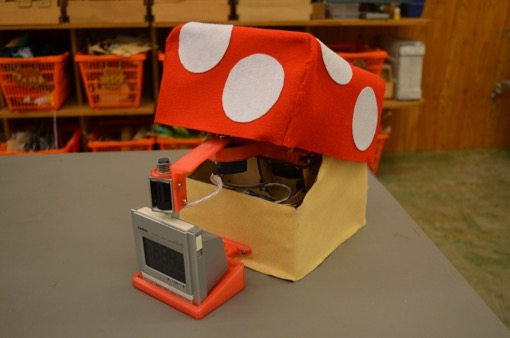#### “魅力四射”的机器人制作【下篇】

2.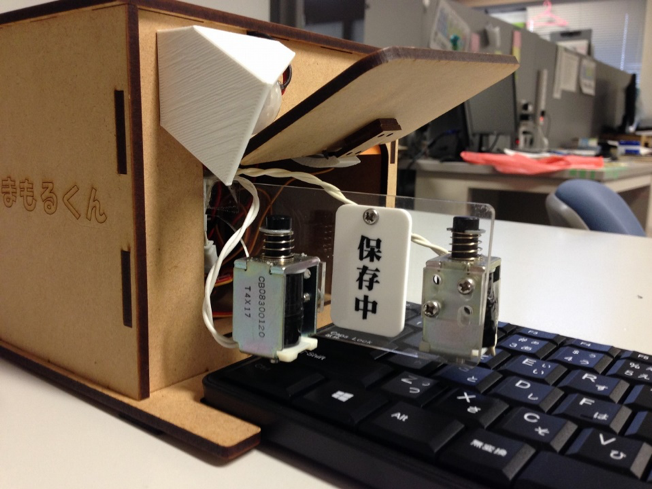#### “魅力四射”的机器人制作【上篇】

3.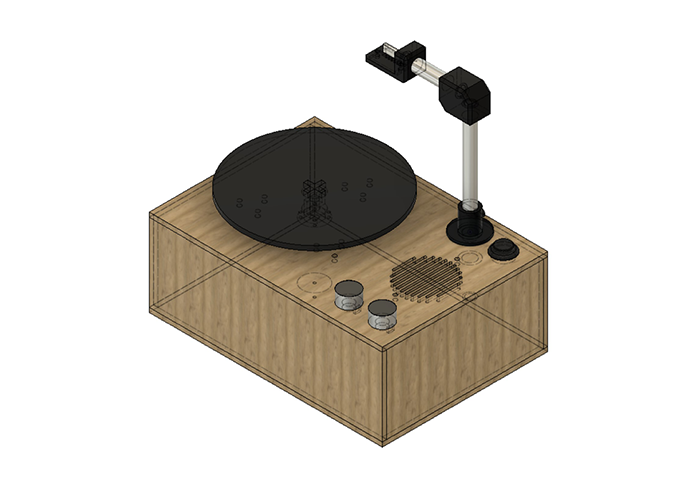#### 用Arduino和TOF距离传感器制作甜甜圈播放器【后篇】

4.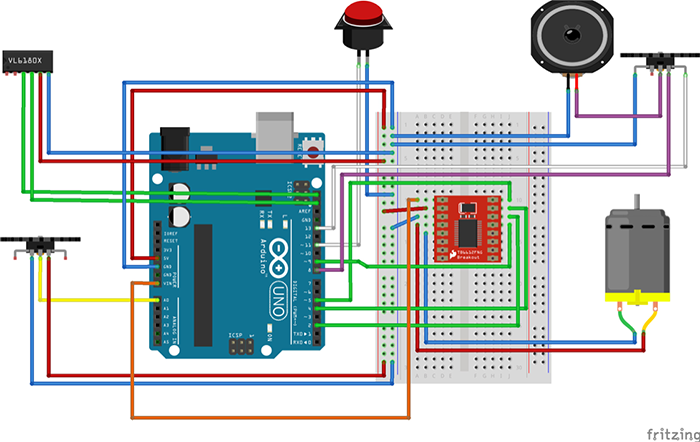#### 用Arduino和TOF距离传感器制作甜甜圈播放器【前篇】

5.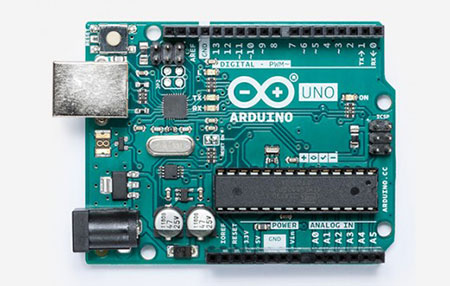#### Arduino电子制作总结！基于微控制器的电子制作基础之基础篇

6.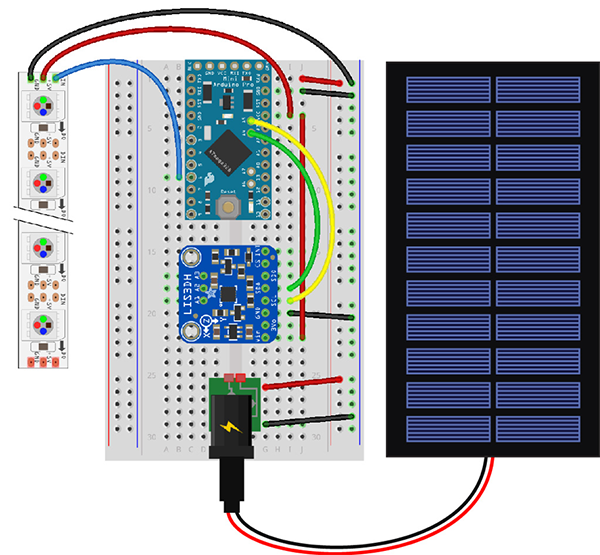#### 用Arduino制作的太阳能电池板供电数字养殖箱【前篇】

7.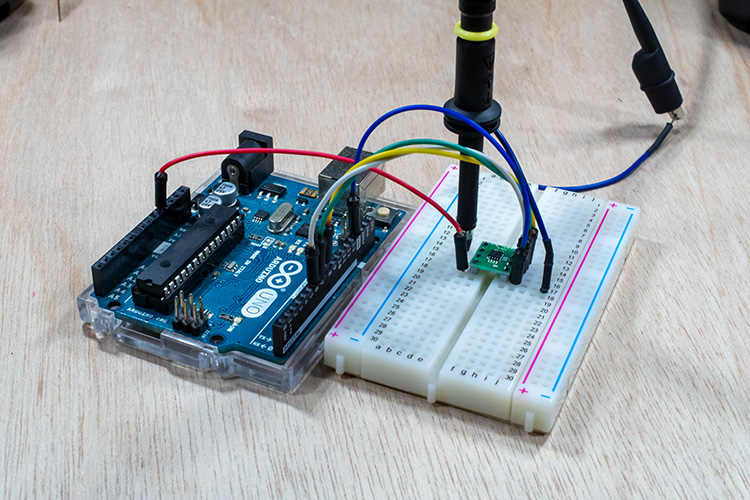#### 使用D/A转换器灵活控制电压并使用Arduino输出模拟信号的方法

8.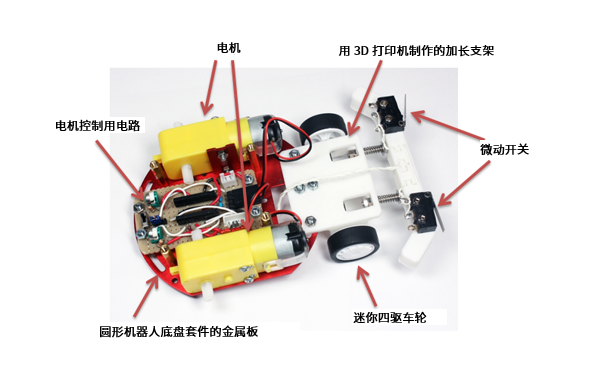#### 在最后一刻停下来！用Arduino和距离传感器制作小鸡赛车！（第3篇•最终篇）

9.